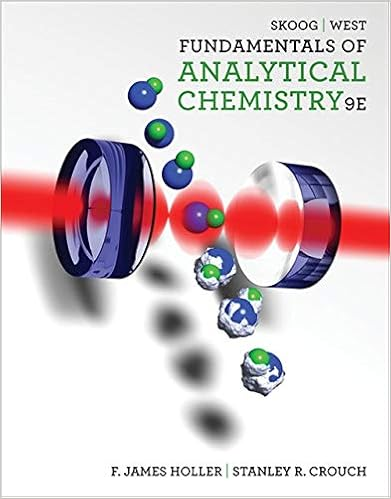# Chm12-2 Practice Exercise 1 Questions.docx - 1 A...

• Homework Help
• 13
• 100% (2) 2 out of 2 people found this document helpful

This preview shows page 1 - 4 out of 13 pages.

##### We have textbook solutions for you!
The document you are viewing contains questions related to this textbook.The document you are viewing contains questions related to this textbook.
Chapter 12 / Exercise 12-24
Fundamentals of Analytical Chemistry
Skoog/WestExpert Verified
1.A temperature of 316.23C is equivalent to:A.43.08KB.143.08 KC.689.38KD.589.38 KE.none of these
1 points QUESTION 21.A sample of nitrogen occupies 200. mL at 27C when the pressure is 0.500 atm. What volume would it occupy at standard conditions?
1 points QUESTION 31.What volume of NO (STP) can be produced by dissolving 62.1 grams of lead in nitric acid?2.3Pb + 8HNO33Pb(NO3)2+ 2NO + 4H2O
1 points QUESTION 41.A 300.-mL sample of hydrogen, H2, was collected over water at 21C ona day when the barometric pressure was 748 torr. What mass of hydrogen is present? The vapor pressure of water is 19 torr at 21
##### We have textbook solutions for you!
The document you are viewing contains questions related to this textbook.The document you are viewing contains questions related to this textbook.
Chapter 12 / Exercise 12-24
Fundamentals of Analytical Chemistry
Skoog/WestExpert Verified
1 points QUESTION 51.A sample of oxygen occupies 47.2 liters under a pressure of 1240. torr at 25C. What volume would it occupy at 25C if the pressure were decreasedto 730. torr?A.29.3 B.27.8 LC.80.2 LD.32.3 LE.47.8 L
L
1 points QUESTION 61.Ammonia, NH3, is used to as fertilizer and as a refrigerant. What is the new pressure if 25.0 g of ammonia with a volume of 750 mL at 1.50 atm is expanded to 7.50 L at constant temperature?
1 points QUESTION 71.A 75 mL sample of CO2is collected over water at 25C when the atmospheric pressure is 1.05 atm. How many moles of CO2are present? The
1 points QUESTION 81.The most significant intermolecular forces of attraction between individual nonpolar atoms or molecules in the gas phase are ____ attractions.
1 points QUESTION 91.Given the following van der Waals constants "a", which gas would behave leastlike an ideal gas?A.3.59 L2-atm/mol2B.0.244 L2-atm/mol2C.0.034 L2-atm/mol2D.1.39 L2-atm/mol2E.4.17 L2-atm/mol2
1 points QUESTION 10
•••